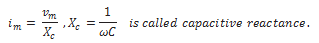• An ac voltage v = vm sinωt applied to a pure inductor L, drives a current in the inductor i = im sin(ωt – π/2), where im = vm/XL.
• XL = ωL is called inductive reactance. The current in the inductor lags the voltage by π/2. The average power supplied to an inductor over one complete cycle is zero.
• An ac voltage v = vm sinωt applied to a capacitor drives a current in the capacitor: i = im sin(ωt + π/2) Here,• The current through the capacitor is π/2 ahead of the applied voltage. As in the case of inductor, the average power supplied to a capacitor over one complete cycle is zero.